## Csound Score Generation and Granular Synthesis with CMask

Andre Bartetzki

STEAM - Studio für elektroakustische Musik, Hochschule für Musik, Berlin, Germany

The program CMask is intended as a handy tool for composers. It provides the Csound user with functions for the global controlling of thousands of score events. This article describes some methods for the generation of Csound score files by stochastic means.

### I. Basics

The score generation process can be considered as a scheme shown below. Every note pfield pn, i.e. instrument number p1, start time p2, duration p3 and all other parameters p4, p5 ... will be calculated according to its own realization of this scheme.
The blue moduls are number generators: constant, list, segment function, random and oscillator. They can be used only alternatively, either RND or OSC or SEG and so on.
The grey moduls are modifier: tendency mask, quantizer and accumulator. QUANT and ACCUM are optional, they can be bypassed as the red lines show.
The result of this process is a value for the note parameter.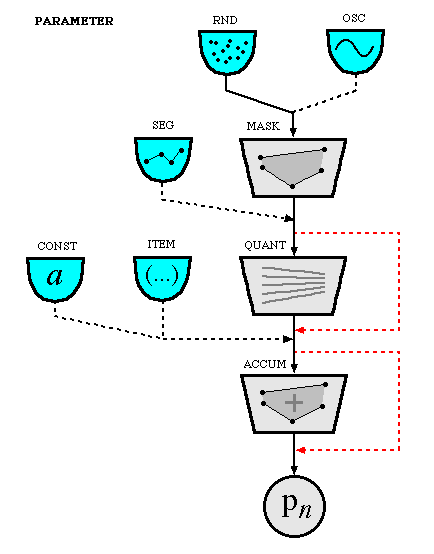A group of note parameters forms a field. A field is valid for a certain time span.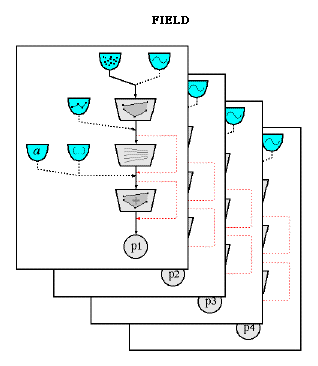The description of one or more fields is contained in a text file.
This file acts as input for CMask, similar to the .orc and .sco files for Csound.
CMask's output is a score file that can be used directly for the sound generation with Csound.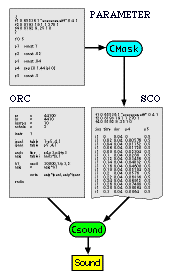The next sections introduce some of the most important principles used in CMask.

#### Probability Control

The first stage in the score generation process is the generation of "raw" numbers for a note statement pfield. "raw" means that they are designated for a further processing as the scheme above indicates. One of the possible number generators is the RND module. Its random values follow a certain probability distribution. These distributions are also implemented in Csound as unit generators (the `xlinrand` family) and GEN routines (`GEN21`). The simplest of them is the uniform distribution. Every value in the random generators range {0,1} has the same probability to appear, like the six sides of a dice.

``rnd uni``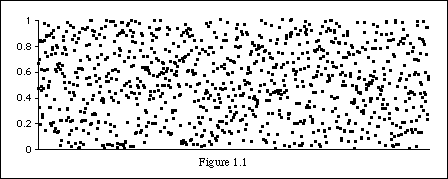From a global point of view there is no preferred area in the whole range from a certain minimum to a maximum ( 0.0 to 1.0. in case of figure 1.1). Every normal random function in a programming language should have a similar behavior as well as numbers in lotto and roulette. Another word for random numbers in a succession is noise. Uniform distribution corresponds to white noise. The rand unit generator in Csound produces uniform distributed numbers and is therefore a white noise generator.
A more important distribution for describing natural processes by mathematical statistics is the gaussian or normal distribution. It prefers numbers in the middle of a range. Two values mark this middle area: the mean and the standard deviation. The mean is the number in the range where we have the most frequent values. The standard deviation ist the width around the mean. Figure 1.2 shows the gaussian distribution (solid line) and the variation of both parameters (dotted lines): a smaller mean and a smaller deviation.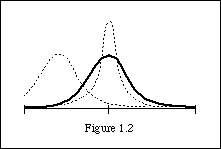A gaussian random generator with a mean of 0.5, a standard deviation of 0.2 and a range of 0.0 to 1.0 produces values shown in figure 1.3.

``rnd gauss .2 .5``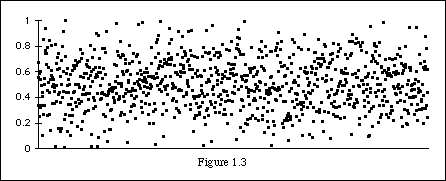The numbers in the next picture was generated by the same gaussian generator except for the mean which was set down to 0.2.

``rnd gauss .2 .2``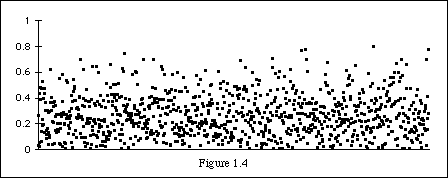Several probability distributions are available in Csound and CMask: uniform, linear, exponential, gaussian, beta and others. For a detailed desription of the various distributions refer in [a]. Some of them have parameters like the gaussian distribution. The exponential distribution for example, which is characterized by a dominance at the lower end of the range, is controlled by a value lambda. A higher lambda describes a stronger dominance of low values. A lambda near 0 results in a uniform-like distribution. If we change that lambda value during the generation of successive random values we can control the the relative appearence of lower values over the time. This is comparable to a low pass filter with a variable cut-off frequency. Below is shown the output of an exponential distribution generator (figure 1.5), whose lambda goes from 0.1 to 3.0 at the middle of the process and finally back to 0.1 (figure 1.6).

``rnd exp (0 0.1 5 3.0 10 0.1)``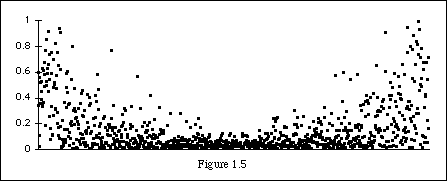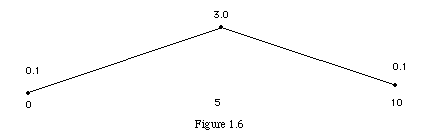Every random generator in CMask is limited to the range {0,1}. In the next score generation stage the numbers within this range will be mapped to a new range required for real Csound note pfields, for example frequencies between 400Hz - 1000 Hz or durations between 0.1 - 2.0 seconds. This is done by tendency masks.

A mask is a time variant range, which is described by the lower and the higher limit. The limits itself can be constant or time-variant. The mapping from the random range {0,1} to the mask's range is a simple linear function: 0 goes to the lower limit of the mask, 1 to the upper limit.
Let's take a uniform distribution and a mask with a constant lower limit of 200 and a constant higher limit of 400. If we regard these values as frequencies and use the numbers in audio oscillators, we get random pitches approximately between g3 and g4:

``mask 200 400``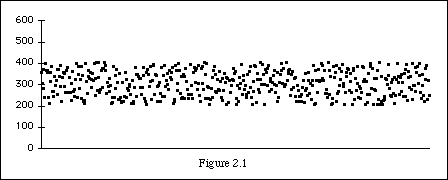In the next example we have a real tendency in the mask. The lower limit goes from 200 to 600, the higher limit goes from 400 to 600 too. That means the range will be smaller and higher over time. At the end, where the limits have the same value, all the random values are mapped onto 600 - there is no other number possible than this number at this point!

``mask [200 600] [400 600]``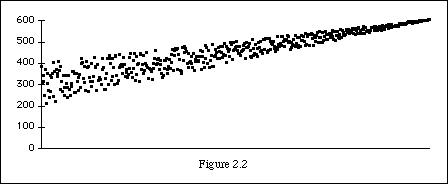Figure 2.3 shows the same higher limit as in figure 2.2. But the lower limit now consists of three points: it starts at 200, goes to 10 at the middle and ends again at 600.

``mask (0 200 5 10 10 600) [400 600]``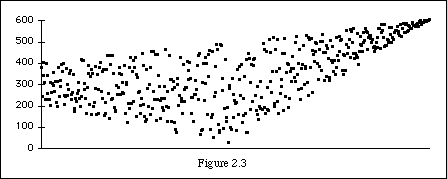Each boundary can be described by an unlimited number of points. Every point is a pair of a time value and a function value. The function values between the specified points will be computed by interpolation, like in the linseg and expseg unit generators. The normal case is a linear interpolation, but there are others. Here we have again the same mask with a negative exponential interpolation for the lower limit:

``mask (0 200 5 10 10 600 ipl -1) [400 600]``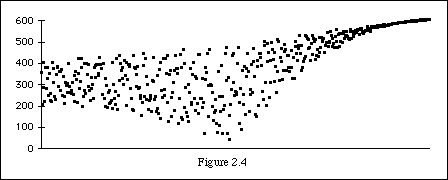More on tendency masks in [b][c].

#### Time

We have seen that a tendency mask is a time-dependent random range. Every note (i-statement) in Csound has its onset time `p2`. This is the time at which CMask evaluates the current range of each pfield `p1...pn`. The onset time p2 itself can also be generated by a random function and a mask. The `p2` value in a Csound score is normally an absolut time measured in seconds (if the tempo is 60). But in CMask it is necessary to handle p2 as the time difference or interval between two successive events.
Figure 3.1 shows the first values of p2 generated by a uniform random function in a constant range from 0.01 to 1 seconds (red diamonds). The next onset time (black dots) is always the current time plus the random value.``````p2 	rnd uni

The first time is 0 seconds. The generated value at this time is by chance 0.75. Therefore, the next time is 0.75. At 0.75 the random value is about 0.5, the new time is 0.75+0.5=1.25. And so on.

Now, we look at an almost complete example. Apart from random functions and masks there are other possibilities for determining pfield values: constants and segment functions.among other things.
A group of events, that share the same masks, random functions etc., is called a field. The first line in the example below is the field header with start and end time of the field. `p1`, the instrument number, is set to 1, that is every note in this field belongs to instrument 1. The onset time or the rhythm value comes from a uniform distribution between 0.01 and 1.0. `p3` (duration) and `p4 `(a frequency ?) remain constant. `p5` is in a random range between 100 and 200. `range` is a shorthand for uniform distribution and an unchanging mask range. `p6` is generated by a segment or break-point function with linear interpolation. One can regard seg as a mask, whose lower and upper bounds are the same. The list after seg contains 3 points: 150 at 0, 300 at 5 and 10 at 10 seconds.

``````f	0 10

p1	const 1
p2	rnd uni
p3	const .1
p4	const 100
p5	range 100 200
p6	seg  (0 150 5 300 10 10)``````

The diagram shows one possible output of this field for `p4` (red diamonds), `p5` (green dots) and `p6 `(blue triangles) over the 10 seconds. Note, that the three pfield values have always the same time. This is the current onset time. You can see `p2` as the gap between these times.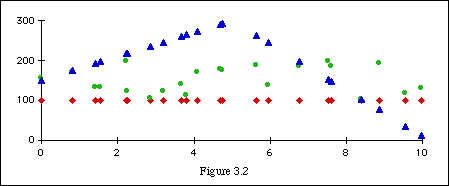Another Example, now with a time-dependent range for `p2.` The brackets [] are a special notation for a segment function that has only two points, one at the start time of the field and one at the end. The number after the word ipl is the optional interpolation value. The default is 0 - this is linear. 1 results in a slightly exponential rise or decay. The mask for `p4` has three points for both limits.

``````f	0 10

p1	const 1
p2	rnd uni
mask [.01 .5 ipl 1] [.02 1  ipl 1]
p3	const .1
p4	rnd uni
mask (0 100 2 500 6 300) (0 1000 2 500 6 1000)``````

Figure 3.3 depicts the mask for the rhythm values in red lines. These values are very small at 0 seconds and rise to {0.5, 1.0} at the end. The gray lines mark the mask of `p4`, the dots are the random values. Note, that both last points of the mask are at the 6th second, their values keep constant from now on. CMask generally uses the first value before its time and the last value after its time - this is different to the behavior of the line and expon modules in Csound were we have an extrapolation of the funtion.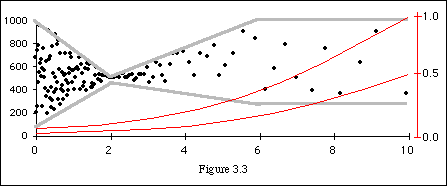#### Quantization

The next optional step in the score generation process is the quantization. Three dynamical parameters determine this quantization: the quantization interval, the offset and the strength. The interval or the quantum can be regarded as the width of a grid. In figure 4.1 we see the output of a random generator in a constant mask between 100 and 400 after a subsequent quantization with an interval of 70 and a maximum strength of 100%. The multiples of 70 that fall in the range {100,400} are 140, 210, 280 and 350. Figure 4.2. shows the same interval but only a 70% strength.

```range 100 400 quant 70 1```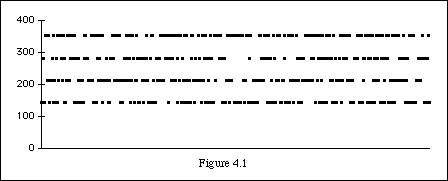```range 100 400 quant 70 .7```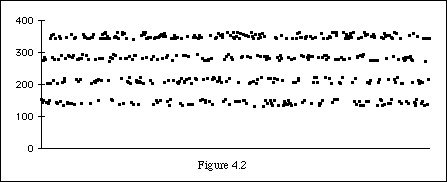The strength is a kind of attraction. 0% means no quantization at all. 50% means that every random number is attracted to the half distance between this random value and the next grid value. 100% means that all numbers go to their next grid point. The strength and the other quantization parameters can be given as a constant or as a segment function. Example 4.3 results from a dynamic strength that goes from 0 to 1.

```range 100 400 quant 70 [0 1]```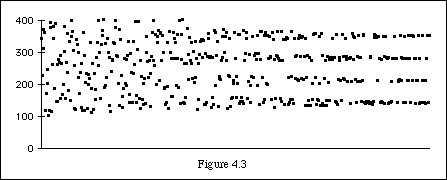The offset is a shift of the quantization grid. With an interval of 70 the grid is ... -140 -70 0 70 140 210 ... . With an offset of 20 we have ... -120 -50 20 90 160 230 ... . The next picture shows a linear rising offset between 0 at start and 70 at the end. The result is a grid wrapped around the masks boundaries. If the values were frequencies we would hear a shepard grain stream.

```range 100 400 quant 70 .9 [0 70]```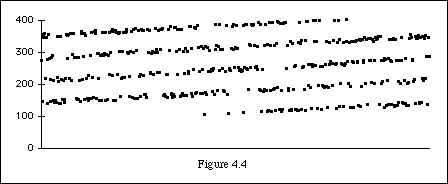An elaborated concept of quantization - the sieves - can be found in .

#### Random Walks

The last step in the score generation procedure is an optional accumulation of the up to now generated and modified numbers. This is done by a simple addition of all values to an inital value (0 by default). The example shown in the next figure is generated by the following code fragment:

``````p4	rnd uni
accum on init 200``````

The uniform random values was mapped into {-50,50} and then added to 200. The result looks like the walk of a drunken man/woman. Another name for this thing is brownian motion or 1/f^2 noise.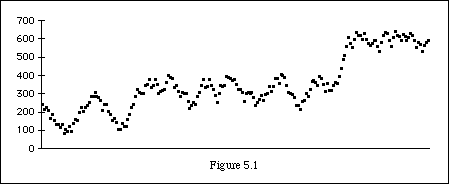An accumulator can also have its own boundaries, like a mask, in order to prevent too small or too high values. Here we have constant limits at 100 and 400. One can see how the drunken dots knock against the upper wall.

``accum limit 100 400 init 200``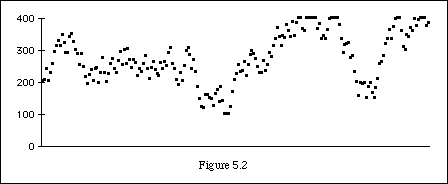There are 2 other accumulation modes. The wrap mode handles the lower and the upper bounds as they were sticked together like a (cylindrical) tube. That means that a value above the upper limit, for example 420, comes out above the lower bound, in this case at 120.

``accum wrap 100 400 init 200``
`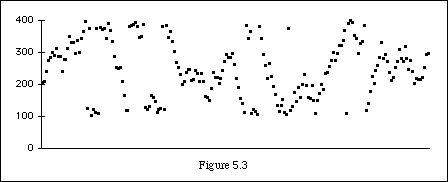`

### II. Granular Techniques

This section describes some applications of CMask and the instrument designs belonging to them.

#### Textures

The term texture stands for a musical object that has a comparatively weak inner structure. Its counterpart is an object that have a strong structure like a melody, a theme, a rhythm. Natural sound textures can be found were many similar acoustic sources sound together: applause, public chatter, crowds of birds, bees or mosquitos, stones in an avalanche, the tuning of the orchestra ... .

The first example uses a simple FM bell. Instrument 1 has 2 additional parameters: the base frequency and a panorama value between 0 (left) and 1 (right).

``````;;; texture1.orc -----------------------
sr	=	44100
kr	=	4410
nchnls	=	2

instr	1

;p4 frequency
;p5 pan (0...1)

ipanl	table	1-p5,1,1
ipanr	table	p5,1,1
k1	expon	1,p3,.01
a1	foscil	k1*4500,p4,1,2.41,k1*6,2
outs	a1*ipanl, a1*ipanr

endin

;;; texture1.orc -----------------------``````

The CMask parameter file `texture1` contains the necessary ftables for panorama crossfade and the sine wave. The longer field (`f 0 30`) consists of three processes: rhythms values gets smaller (`p2`), the frequency mask gets wider (`p4`) - the frequencies itself are bound to an overtone series build on 100 Hz - and the stereo image gets broader (`p5`). The mapping value 1 in `p4` guarantees more lower frequencies. The shorter field (`f 31 33`) describes a ritardando with random frequencies between 300 and 400 Hz and a motion from left to right.

``````;;; texture1 ---------------------------
{
f1 0 8192 9 .25 1 0
f2 0 8193 10 1
}

f	0 30

p1 	const 1
p2 	rnd uni
mask [.01 .002 ipl 0] [.1 .01 ipl 0]
p3 	range .5 1
p4 	rnd uni
mask [860 80 ipl -1.2] [940 2000 ipl 1] map 1
quant 100 .9 0
p5 	mask [.4 0] [.6 1]

f	31 33

p1 	const 1
p2 	seg [.08 .8 ipl 2]
p3 	seg [.1 2]
p4 	range 300 400
p5 	seg [0 1]

;;; texture1 ---------------------------``````

CMask produces with this parameter file a Csound score file `texture1.sco` with about 1370 events in the first field and 15 events in the second field.

The next example is based on sound samples of doors. It has 4 parameters: a transposition factor, a ftable number - that is actually the sound file number - , the panorama value and a reverb balance. The global result is a gesture similar to the previous example.

``````;;; doors.orc -----------------------
sr	=	44100
kr	=	4410
nchnls	=	2

garev	init	0

instr	1

;p4 transposition (1=normal)
;p5 table number (1...6)
;p6 pan (0...1)
;p7 dry/wet (0...1)

ipanl	table	1-p6,10,1
ipanr	table	p6,10,1
k1	expon	.5,p3,.01
a1	loscil	k1,p4,p5,1,0,0,2
a1	linen	a1,0,p3,.05
garev	=	garev + a1*p7
a2	=	a1*ipanr
a1	=	a1*ipanl
outs	a1*(1-p7*p7), a2*(1-p7*p7)

endin

instr 99

krev	expon	.03,p3-4,5
a1	reverb2	garev,krev,.2
a2	reverb2	garev,krev*1.1,.21
outs	a1/2,a2/2

garev	=	0

endin

;;; doors.orc -----------------------``````

The `doors` parameter file begins with the GEN01 sound file tables and the panorama function. Instrument 99, the reverberator, sounds all the time. Rhythm values for `instr 1` can be chosen from the range {0.01, 0.1}. The beta distribution for `p2 `ensures that we get small groups or rather gaps between events like stumbling. The durations increase from 300 msecs to 2 seconds. `p4` - the transposition factor - goes from a range {3,5} to {0.1,0.2}. This means that we have values about 2 octaves higher at start and finally more than 2 octaves lower. `p5` selects the ftable from a range between 1 and 6 with a precision of 0, i.e. as integer. The reverb balance is controlled by `p7`.

``````;;; doors --------------------------
{
f1 0 0 -1 "door1.aiff" 0 4 1
f2 0 0 -1 "door2.aiff" 0 4 1
f3 0 0 -1 "door3.aiff" 0 4 1
f4 0 0 -1 "door4.aiff" 0 4 1
f5 0 0 -1 "door5.aiff" 0 4 1
f6 0 0 -1 "door6.aiff" 0 4 1
f10 0 8192 9 .25 1 0

i99 0 23
}

f 	0 20

p1 	const 1
p2 	rnd beta .05 .1
mask (12 .01 18 .2) (12 .1 18 1)
p3 	seg [.3 2 ipl .4]
p4 	mask [3 .8 ipl .4] [5 1.2 ipl .4]
p5 	range 1 6
prec 0
p6 	range 0 1
p7 	seg (2 0 18 .5 ipl 1)

;;; doors --------------------------```

```

#### Sound file granulation

Granular synthesis uses mostly sound files instead of synthetic wave forms and is therefore actually a mix of sampling and resynthesis. Granulation is the term for cutting a sound into small pieces - the grains. Their duration is normally very short: about 5 to 50 msecs. This time range marks also the transition area from low pitches to fast tempi. (It is not by chance that this is also the domain of frame rates in movies and vertical frequencies in monitors.) The sound quality of a single grain is among other things determinated by its envelope. There are many different envelopes or window functions for granular synthesis: real-time systems use often triangles, trapezoids or simple rectangles, but to have a cleaner sound it is advisable to use smoother functions like hamming or hanning windows, splines or phase shifted sine waves. Figure 6.1 shows a spline function.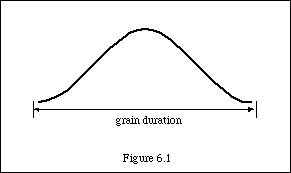The granulation might be considered as a meta-sampling process: every grain contains a small sample from the whole sound. First we take same grains - like snapshots with a camera - and then we rearrange them in any order and distance. For many applications it is necessary that a sequence of grains has a proper overlap. Depending on the envelope shape it is then possible to reconstruct the original soundfile. To do this, some conditions have to meet: the sum of the grain envelopes must have always the same amount at every time, and size, distance and order of the grains have to be the same as before the rearrangement.Now, there are unlimited possibilities to transform a sound file by granulation. We have many changeable parameters such as grain duration, grain envelope and amplitude, grain distance or overlap and grain order, as well as all the other traditional transformation techniques like filtering, modulation, positioning in space (angle, distance and reverberation) and so on.
A simple time stretching technique, for example, uses the overlap factor as transformation parameter. If we rearrange the grains in the same order but with a half overlap factor we will get a sound that takes the double time. In order to prevent gaps in granular time stretching it is better to have a higher overlap while scanning the sound file and a normal overlap of 2 while rearranging. To shorten a sound we have to multiply the overlap factor or rather shorten the distance between the grains. The principle of stretching is shown in figure 6.3. The first row is the original order, the second is the new order.For further studies on granular techniques see [c].

In order to granulate a sound file with Csound and CMask we need some preparation! Since we let CMask compute the grains as score notes it is advisable to load the complete sound file in a GEN01 table instead of using `soundin` , in order to prevent thousands of hard disk accesses during the sound generation process. Normally we can use GEN01 ftables only in conjunction with `loscil` if the sound file size is unequal to a power of 2. But `loscil` is not very useful for our purposes, so we have to lengthen the sound with silence or cut to the nearest power of 2. For example:

``f1 0 262144 1 "sound" 0 4 1``

If `sr = 44100 `this table contains 5.94 seconds regardless of the original sound file length.
Suppose we want to play a certain part of the ftable 1 at a certain time `p2` for a certain duration `p3`. Then we must have a time pointer or an index to this part. The following code fragment shows a solution. It makes use of `p4` as initial index measured in seconds.

``````andx	line	p4,p3,p4+p3
asig	table	andx*sr, 1``````

The index andx goes from ftable time` p4 `during a time `p3` to ftable time `p3+p4`. The multiplication `andx*sr` converts seconds to sample frames so that we have a raw index. `ksig` provides the envelope and a proper amplitude if the GEN01 table is normalized.

``````ksig	oscil	20000,1/p3,2
out	asig*ksig``````

The corresponding ftable 2 contains for example a phase shifted sine wave with a DC offset as envelope function:

``f2 0 8192 19 1 1 270 1``

The macroscopic organization of the grains is the business of CMask. For a simple constant time stretching we need a constant time advance and duration:

``````p2 	const .02
p3 	const .04``````

The overlap is set by the relation of `p3` to `p2` - in this case the factor is 2. The grain pointer `p4` goes over the ftable's size with a constant speed:

``p4 	seg [0 5.9]``

Now, let's have a look at a complete example.

``````;;; axa1.orc --------------------------
sr	=	44100
kr	=	4410
nchnls	=	2

instr	1

;p4 grain pointer (in seconds)
;p5 pan (0...1)

ipanl	table	1-p5 ,4,1
ipanr	table	p5 ,4,1
andx	line	p4,p3,p4+p3
asig	table	andx*sr,1
k1	oscil	30000,1/p3,2
asig	=	asig*k1
outs	asig*ipanl, asig*ipanr

endin

;;; axa1.orc --------------------------``````

The sound file used here is speech in a fictious language:

``````;;; axa1 ------------------------------
{
f1 0 65536 1 "axaxaxas.aiff" 0 4 1 ; 65536 samples @ 44.1 = 1.4861 sec
f2 0 8193 19 1 1 270 1	; grain envelope
f4 0 8192 9 .25 1 0	; pan function
}

f 0 5

p1 	const 1
p2 	const .02
p3 	const .04
p4 	seg [0 1.44]
p5 	const .5

;;; axa1 ------------------------------``````

The interpolation exponent in the segment function for `p4` is set to 0 by default. This means that `p4` follows a linear function. The value of `p4` begins at 0 and ends after 5 seconds (the field's duration) at 1.44. Therefore we have a stretch factor of `5/1.44=3.47`. But if we change that value to -2, for example, we get a nonlinear function: fast rising at the beginning and very slow in the end.

``p4 	seg [0 1.44 ipl -2]	``

That is, the scanning overlap factor goes from small to large and we get a dynamical time stretching: from fast to slow like a ritardando.
The reverse principle - an accelerando effect - is shown in figure 6.4: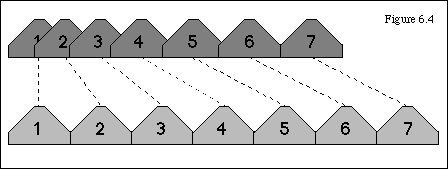With a little change in the definition for `p4` it is easy to get a texture of grains instead of time stretching:

``p4 	range 0 1.44	``

This texture has a constant density because `p2` and `p3` are constant. To get a more random texture we can write the following for example:

``````p2	mask .005 .1 map 1
p3	range .04 .2	``````

Figure 6.5 shows the scheme of a random granular texture: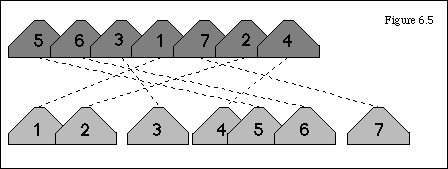A mean between dynamic timestretching and a complete random structure can be found in random walks. The mask produces time steps between 2 and 50 msecs and the accumulator adds them together up to a maximum of 1.4. The wrap mode causes that the next steps starts again from 0.

``````p4 	mask .002 .05 map 1
accum wrap 0 1.4``````

The last example uses an instrument similar to the previous `instr 1`. Two small changes happened here:

``````andx	line	p4,p3,p4+p3*p6
asig	tablei	andx*sr,1``````

Parameter `p6` is a factor for transposition. Supposing `p6 = 2` the index goes from `p4` over the time `p3` to a position that has the double distance compared to `p3`. The grain comsumes now a part of the sound file that is larger than its own duration. In case of `p6=2` this is the double speed or a transposition to 1 octave higher. With this technique we can transpose a sound without changing its length. If `p6` will be very small it is advisable to use an interpolating table to reduce the noise level.
This example is a slightly random time stretching partly with quantized transposition.

``````;;; whisper.orc --------------------------
sr	=	44100
kr	=	4410
nchnls	=	2

instr	1

ipanl	table	1-p5 ,4,1
ipanr	table	p5 ,4,1
andx	line	p4,p3,p4+p3*p6
asig	table	andx*sr,1
k1	oscil	8000,1/p3,2
asig	=	asig*k1
outs	asig*ipanl, asig*ipanr

endin

;;; whisper.orc --------------------------

;;; whisper ------------------------------
{
f1 0 262144 1 "whisp.aiff" 0 4 1 	; = 5.94 sec
f2 0 8192 19 1 1 270 1			; grain envelope
f4 0 8192 9 .25 1 0			; pan function
}

f 0 60

p1 	const 1
p2 	mask (0 .0005 37 .007 60 .003) (0 .003 37 .15 60 .005)
p3	mask [.3 .02] [.7 .04]
p4	seg [0 5.9]
p5 	range 0 1
p6	mask (0 .3 25 1 40 .7) (0 2 4 1 25 1.2)
quant .3 (0 0 25 .9 30 0 45 .9 55 0) (40 0 45 1.5 55 0)

;;; whisper ------------------------------``````

CMask runs currently only on Macs, PowerMacs, SGI IRIX 5.3. and WIN95
Program, manual and examples are available at:

For further information about CMask and other utilities as well as computer music activities in Berlin check out my website.

http://www.kgw.tu-berlin.de/~abart/

### References

 Ames, Ch. 1991. "A Catalog of Statistical Distributions..." Leonardo Music Journal 1(1)
 Dodge, Ch. / Jerse, Th. A. 1985. Computer Music Collier Macmillan, London
 Lorrain, D. 1980. "A Panoply of Stochastic 'Cannons'." Computer Music Journal 4(1)
 Roads, C. 1991. "Asynchronous Granular Synthesis." in Representations of Musical Signals, MIT Press
 Roads, C. 1985. "Granular Synthesis of Sounds." in Foundations of Computer Music, MIT Press
 Truax, B. 1988. "Real-time Granular Synthesis with a Digital Sound Processor." Computer Music Journal 12(2)
 Wishart, T. 1994. Audible Design Orpheus the Pantomime
 Xenakis, I. 1992. Formalized Music Pendragon Press

### Software

[a] Castine, P. Litter Package (Externals for MAX)
[b] Koenig, G.M. Project I, Project II
[c] Truax, B. POD programs
[d] Xenakis, I. Stochastic Music Program

March 1997, Andre Bartetzki @ STEAM, HfM Berlin